# SurfacePattern: a Mathematica package for surface atom and ion traps

by Roman Schmied, 2009-2018

## Description of operation

### "forward" problem

Electrostatic and magnetostatic problems involve solving the Laplace equation with Dirichlet boundary conditions. In the specific case where this boundary condition is given on the entire xy plane, the Mathematica package SurfacePattern can solve such problems exactly and give analytic results for the resulting three-dimensional electric and scalar magnetic potentials, as well as their first, second, and third (up to fifth for finite problems) spatial derivatives. Specifically, if a potential function f(x,y) is given on the xy plane, then the unique potential F(x,y,z) can be calculated which satisfies F(x,y,0)=f(x,y) as well as ΔF(x,y,z)=0 for z>0. Additionally, the constraint that F(x,y,H)=0 for a certain "cover plane" height H can be imposed.

With the SurfacePattern package, the function f(x,y) can be defined in two ways:

• Periodic functions (wallpaper symmetry group p1): the user specifies two lattice vectors (defining the unit cell) as well as the value of f(x,y) on an n×n grid of equal-size parallelograms which cover one unit cell.
• Arbitrary functions: the user assembles f(x,y), and therefore F(x,y,z), by adding up polygon-shaped and disk-shaped features. For a polygon, specifying a list of points in the xy plane results in an f(x,y)with value 1 inside and 0 outside the polygon. Disk shapes are given by their center and radius.

There are at least three experimental setups where such problems occur, and for which the SurfacePattern package provides assistance. Ref.  shows how they are all mathematically equivalent.

• Surface-electrode ion traps [1,2] consist of a patterned electrode plane, whose different pieces can be connected to different rf or dc electric potentials, in such a way that the resulting three-dimensional electric fields trap ions at specific points in space. In Ref.  I have shown that the unavoidable gaps between electrode pieces are irrelevant, as is the fact that in practice the surface-electrode plane is not infinite in extent. As a result, the electric potential due to this patterned electrode plane can be calculated with the procedure described above, where F(x,y,z) is the electric potential in space and f(x,y) is the electric potential in the electrode plane (i.e., applied to the electrodes).
• Surface magnetic traps , for example of the Ioffe–Pritchard type, are generated by patterned thin films which are permanently magnetized out-of-plane. With f(x,y) taking the role of the surface magnetization strength and F(x,y,z) that of the scalar magnetic potential (defined such that the magnetic field is proportional to its gradient), the magnetic field due to any magnetization pattern can be calculated with the above procedure.
• Magnetic wire traps are very similar to surface magnetic traps, except for the way in which the magnetic fields are generated. Instead of permanently magnetizing surface regions, we can replace their edges by wires which carry a current equal to the surface magnetization strength. This results in exactly the same magnetic fields by the Kelvin–Stokes theorem.

Calculating F(x,y,z) from f(x,y) is neither difficult nor new. What the SurfacePattern package provides is a versatile library of useful algorithms for doing such calculations in practice, particularly in the context of the "backward" problem below.

### "backward" problem

A more interesting and more rewarding problem than the above "forward" calculation is that of finding the optimal function f(x,y) such that the resulting F(x,y,z) satisfies certain constraints. The SurfacePatternpackage can solve this "backward" problem as well.

As for the "forward" problem, the surface where f(x,y) is defined can be taken as periodic or non-periodic. Constraints on F(x,y,z) must be local in space. This means that either F(x,y,z) or its first, second or third (up to fifth for finite problems) spatial derivatives are fixed to certain values at certain points in space; and the SurfacePattern package then finds the unique function f(x,y) which satisfies these constraints while simultaneously maximizing the "strength" of the constraints. This maximization works in the following sense: if, for example, the only constraint on F is that its gradient at the point P= is equal to ∇F(P)=, then the function OptimalFinitePattern finds the pattern f(x,y) which generates a potential with ∇F(0,0,1)=C× with the largest possible value of C, while being bounded (by default) by 0≤f(x,y)≤1. The relaxed Linear Programming method for finding this f(x,y) has been proven to converge extremely rapidly to its unique solution, and the solution is almost binary in that at almost every point  we either find f(x,y)=0 or f(x,y)=1. See Refs. [2,3] for details.

For periodic systems the constraints can also be local in Fourier space.

## How to install

You can either store the SurfacePattern.m package file in the same directory as the Mathematica notebook you are working with, or you can install it through the "File"-"Install..." dialog.

## Usage instructions

For now, the usage instructions are inside the Mathematica package (see downloads). Please study the provided demos which make use of the most important features.

## Frequently Asked/Answered Questions

• Why did you program this in Mathematica, and not in a form which can be used with free software?

I have tried through several freely available Linear Programming solvers and have not found one which gets even close to Mathematica's capabilities for solving the "backward" problem. For reasonable efficiency, we need a primal-dual interior-point (PDIP) solver; simplex solvers are too slow and can give biased results in highly symmetric situations. GLPK's PDIP solver, in particular, cannot handle dense columns, which SurfacePattern requires. If you can show me a free software package with a PDIP algorithm which scales as O(N) in both space and time, I'd be happy to re-implement the SurfacePattern package for it.

Update: Robert Jördens has developed a Python package which implements a similar forward/backward functionality using the free CVXOPT package.

• Can the "backward" procedure of the SurfacePattern package find a solution for my specific problem?

Yes, as long as the constraints do not violate the Laplace equation. The question is, however, how good this solution will be: if the constraints are too complex, then (i) the maximized constant C will be exponentially small, and (ii) the function F(x,y,z) will have a spatial structure which is more complex than what you intended, leading to spurious ion traps (see Ref. ) or magnetic field zeros (see Ref. ) which make the result unusable in practice.

• It's not working, and the documentation is opaque/incomplete/wrong. Help!

Please send me an email and I'll try to help. I especially welcome bug reports, however incomplete. This is a work in progress, and any comments are welcome.

## References

  Roman Schmied: Electrostatics of gapped and finite surface electrodes. New Journal of Physics 12:023038 (2010).  Roman Schmied, Janus H. Wesenberg, and Dietrich Leibfried: Optimal Surface-Electrode Trap Lattices for Quantum Simulation with Trapped Ions. Physical Review Letters 102:233002 (2009).  Roman Schmied, Dietrich Leibfried, Robert J. C. Spreeuw, and Shannon Whitlock: Optimized magnetic lattices for ultracold atomic ensembles. New Journal of Physics 12:103029 (2010).  Mário H. Oliveira and José A. Miranda: Biot–Savart-like law in electrostatics. European Journal of Physics 22:31 (2001).

## How to cite this package

Whenever you publish scientific work which makes use of any component provided on this page, you must add the following references:

 For any application: Roman Schmied, SurfacePattern software package. For any application of the "backward" algorithm: Ref.  above For surface-electrode applications (e.g., surface-electrode ion traps): Refs.  and  above For surface-magnetization applications (e.g., patterned permanently-magnetized thin films or patterned wires): Ref.  above

## Downloads

Below you find the SurfacePattern package code, as well as demo notebooks for the "forward" and "backward" problems, for finite and infinite surface patterns, and for electric and magnetic setups. SEMimages are provided wherever a particular pattern has already been fabricated for experimental use.

(see also Robert Jördens' Python package for an alternative implementation of this functionality)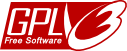Mathematica package for both finite and periodic surface patterns
current version: 2.7.1 (May 28, 2018)
Copyright 2009-2018 Roman Schmied
with contributions by Janus H. Wesenberg

SurfacePattern is free software: you can redistribute it and/or modify it under the terms of the GNU General Public License as published by the Free Software Foundation, either version 3 of the License, or (at your option) any later version.
SurfacePattern is distributed in the hope that it will be useful, but WITHOUT ANY WARRANTY; without even the implied warranty of MERCHANTABILITY or FITNESS FOR A PARTICULAR PURPOSE. See the GNU General Public License for more details.

fileadmin/user_upload/atom_physik/People/Roman_Schmid/SurfacePattern/SurfacePattern_forward_finite_electric.nb.zip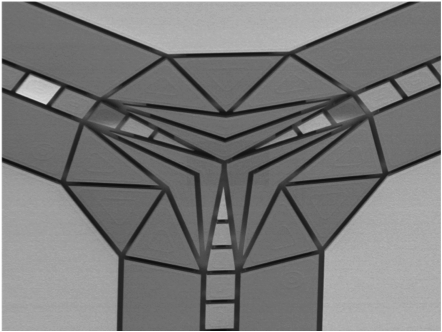image: SNLNISTIARPA 2011

"forward" finite electric demo: calculate the radio-frequency ion pseudo-potential of a switchable Y intersection constructed from polygons in a plane, and show the "guiding tube" for the ion going from one channel to another around a 60° bend

fileadmin/user_upload/atom_physik/People/Roman_Schmid/SurfacePattern/SurfacePattern_backward_finite_electric.nb.zip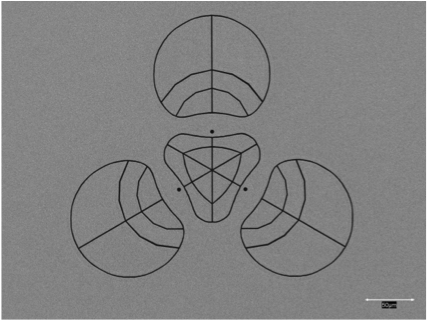image: SNLNISTIARPA 2011

"backward" finite electric demo: optimize the shape of surface electrodes for trapping three ions

fileadmin/user_upload/atom_physik/People/Roman_Schmid/SurfacePattern/SurfacePattern_forward_finite_magnetic.nb.zip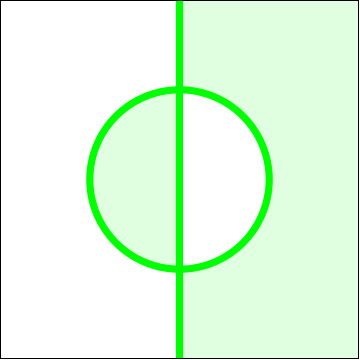"forward" finite magnetic demo: calculate the magnetic field of a few simple patterned magnetized thin films (or wire layouts) described by polygons in a plane: U-wire, Z-wire, loop-wire

fileadmin/user_upload/atom_physik/People/Roman_Schmid/SurfacePattern/SurfacePattern_backward_finite_magnetic.nb.zip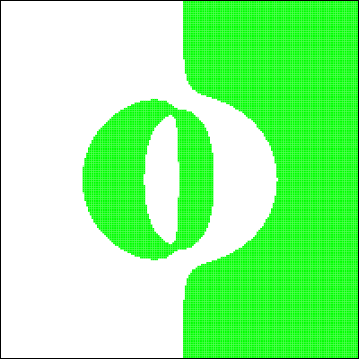"backward" finite magnetic demo: optimize the shape of a surface magnetization pattern for generating one and two Ioffe–Pritchard atom traps

fileadmin/user_upload/atom_physik/People/Roman_Schmid/SurfacePattern/SurfacePattern_forward_infinite_electric.nb.zip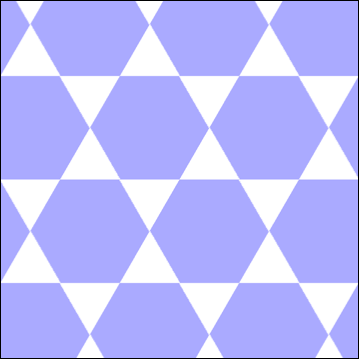"forward" infinite electric demo: calculate the electric potential of an infinite Kagome lattice of surface electrodes

fileadmin/user_upload/atom_physik/People/Roman_Schmid/SurfacePattern/SurfacePattern_backward_infinite_electric.nb.zip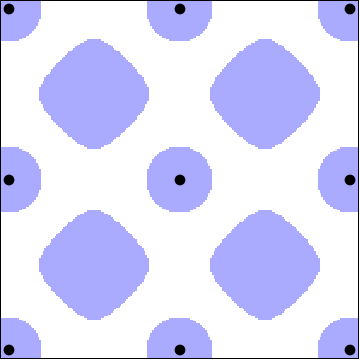pattern from R. Schmied et al.PRL 102:233002 (2009)

"backward" infinite electric demo: optimize the shape of an infinite lattice of rf electrodes for trapping an infinite array of ions in surface Paul microtraps (with dynamic demo)

fileadmin/user_upload/atom_physik/People/Roman_Schmid/SurfacePattern/SurfacePattern_forward_infinite_magnetic.nb.zip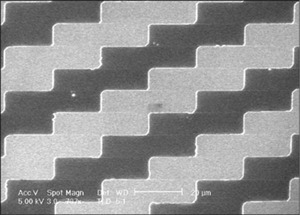pattern from R. Gerritsma et al.PRA 76:033408 (2007)

"forward" infinite magnetic demo: calculate the magnetic field of an infinite periodic surface magnetization pattern

fileadmin/user_upload/atom_physik/People/Roman_Schmid/SurfacePattern/SurfacePattern_backward_infinite_magnetic.nb.zip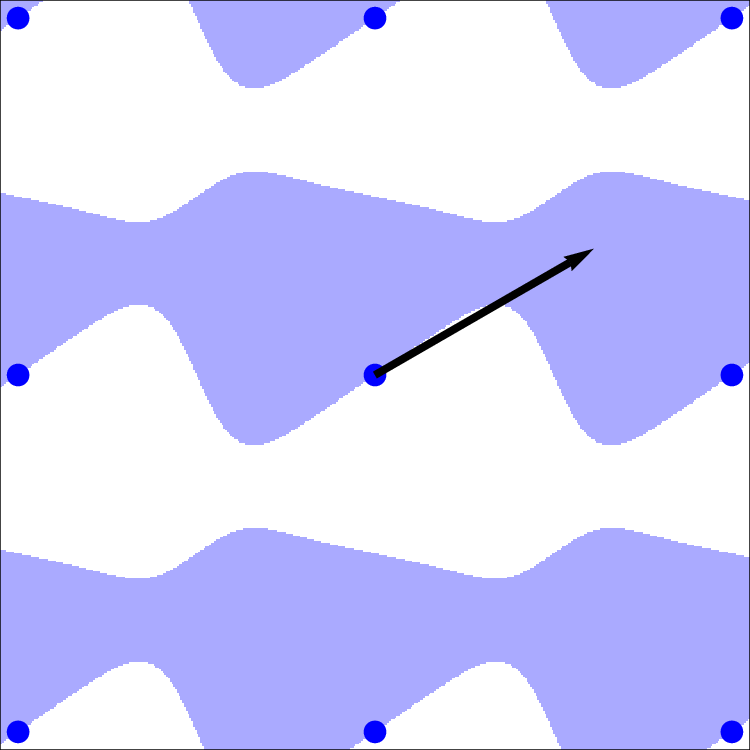pattern from R. Schmied et al.NJP 12:103029 (2010)

"backward" infinite magnetic demo: optimize the shape of an infinite periodic surface magnetization pattern for creating an infinite array of Ioffe–Pritchard traps

last updated on May 28, 2018 by Gianni Buser on behalf of Roman Schmied